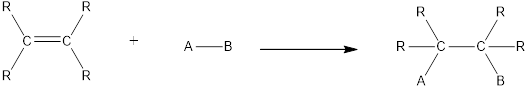Chapter 2, Problem 2.72EP### Organic And Biological Chemistry

7th Edition
STOKER + 1 other
ISBN: 9781305081079

#### Solutions

Chapter
Section### Organic And Biological Chemistry

7th Edition
STOKER + 1 other
ISBN: 9781305081079
Textbook Problem

# Write a chemical equation showing reactants, products, and catalysts needed (if any) for the reaction of ethene with each of the following substances. a. H2O b. Br2 c. HI d. I2

(a)

Interpretation Introduction

Interpretation:

Chemical equation that shows reactants, product and catalyst needed for the reaction of ethene with water has to be written.

Concept Introduction:

Chemical reaction in which an atom or a group of atoms are added to each carbon atom of a carbon‑carbon multiple bond in a hydrocarbon or hydrocarbon derivative is known as addition reaction.

In this reaction no atoms or group of atoms are removed. Instead the unsaturated bond is reduced to saturated bond. A general scheme for addition reaction of alkene can be given as shown below,Hydration is an example of addition reaction. In this reaction, a water molecule is incorporated into the molecules of organic compound. Hydration of alkene results in the formation of alcohol, where one carbon atom gets hydrogen atom added and the other carbon atom gets hydroxyl group added to it. This reaction requires a small amount of sulphuric acid as catalyst.

Explanation

Ethene reacts with water to give product that contains a hydroxyl group. This is an example of addition reaction. Hydration of ethene using water results in the formation of ethanol as product. For this reaction a small amount of sulphuric acid is required as catalyst...

(b)

Interpretation Introduction

Interpretation:

Chemical equation that shows reactants, product and catalyst needed for the reaction of ethene with bromine has to be written.

Concept Introduction:

Chemical reaction in which an atom or a group of atoms are added to each carbon atom of a carbon‑carbon multiple bond in a hydrocarbon or hydrocarbon derivative is known as addition reaction.

In this reaction no atoms or group of atoms are removed. Instead the unsaturated bond is reduced to saturated bond. A general scheme for addition reaction of alkene can be given as shown below,Halogenation reaction is an example of addition reaction. In this reaction, the halogen atoms are added across the double bonds. Chlorination and bromination are the most commonly used halogenation reaction. For halogenation reaction, no catalyst is required.

(c)

Interpretation Introduction

Interpretation:

Chemical equation that shows reactants, product and catalyst needed for the reaction of ethene with hydrogen iodide has to be written.

Concept Introduction:

Chemical reaction in which an atom or a group of atoms are added to each carbon atom of a carbon‑carbon multiple bond in a hydrocarbon or hydrocarbon derivative is known as addition reaction.

In this reaction no atoms or group of atoms are removed. Instead the unsaturated bond is reduced to saturated bond. A general scheme for addition reaction of alkene can be given as shown below,Asymmetrical addition reaction is the one in which two different atoms or group of atoms are substituted across the multiple bond resulting in the formation of product. No catalyst is required for this reaction.

(d)

Interpretation Introduction

Interpretation:

Chemical equation that shows reactants, product and catalyst needed for the reaction of ethene with iodine has to be written.

Concept Introduction:

Chemical reaction in which an atom or a group of atoms are added to each carbon atom of a carbon‑carbon multiple bond in a hydrocarbon or hydrocarbon derivative is known as addition reaction.

In this reaction no atoms or group of atoms are removed. Instead the unsaturated bond is reduced to saturated bond. A general scheme for addition reaction of alkene can be given as shown below,Halogenation reaction is an example of addition reaction. In this reaction, the halogen atoms are added across the double bonds. Chlorination and bromination are the most commonly used halogenation reaction. For halogenation reaction, no catalyst is required.

### Still sussing out bartleby?

Check out a sample textbook solution.

See a sample solution

#### The Solution to Your Study Problems

Bartleby provides explanations to thousands of textbook problems written by our experts, many with advanced degrees!

Get Started

#### Find more solutions based on key concepts